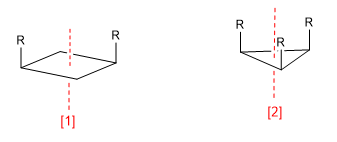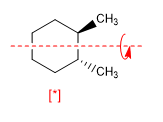A chiral molecule is one that is not superimposable with its mirror image. Symmetry causes molecules to lose their chirality. Thus, the presence of planes of symmetry, inversion centers or alternating axes give rise to achiral molecules.

a) Axis of symmetry (C n )Symmetry axis (C 2 )

Symmetry axis (C 3 )

An axis of symmetry of order m leaves the molecule in a configuration indistinguishable from the initial one when rotating 360/m degrees.

b) Plane of reflection ( s ): Divides the molecule into two equal parts. Every atom of the molecule that is on one side of the plane must have its mirror on the other side.c) Center of symmetry (i): Every atom in the molecule is symmetrical with respect to a point located in the center of the molecule.d) Alternating or improper axis (Sn): The alternating axis consists of two symmetry operations, a turn Cm and a reflection in a plane perpendicular to the axis.A molecule is achiral if it contains an alternating plane, center, or axis of symmetry. The presence of Cn axes does not make a molecule achiral as can be seen in the following example.[*] Chiral molecule with C 2 axis